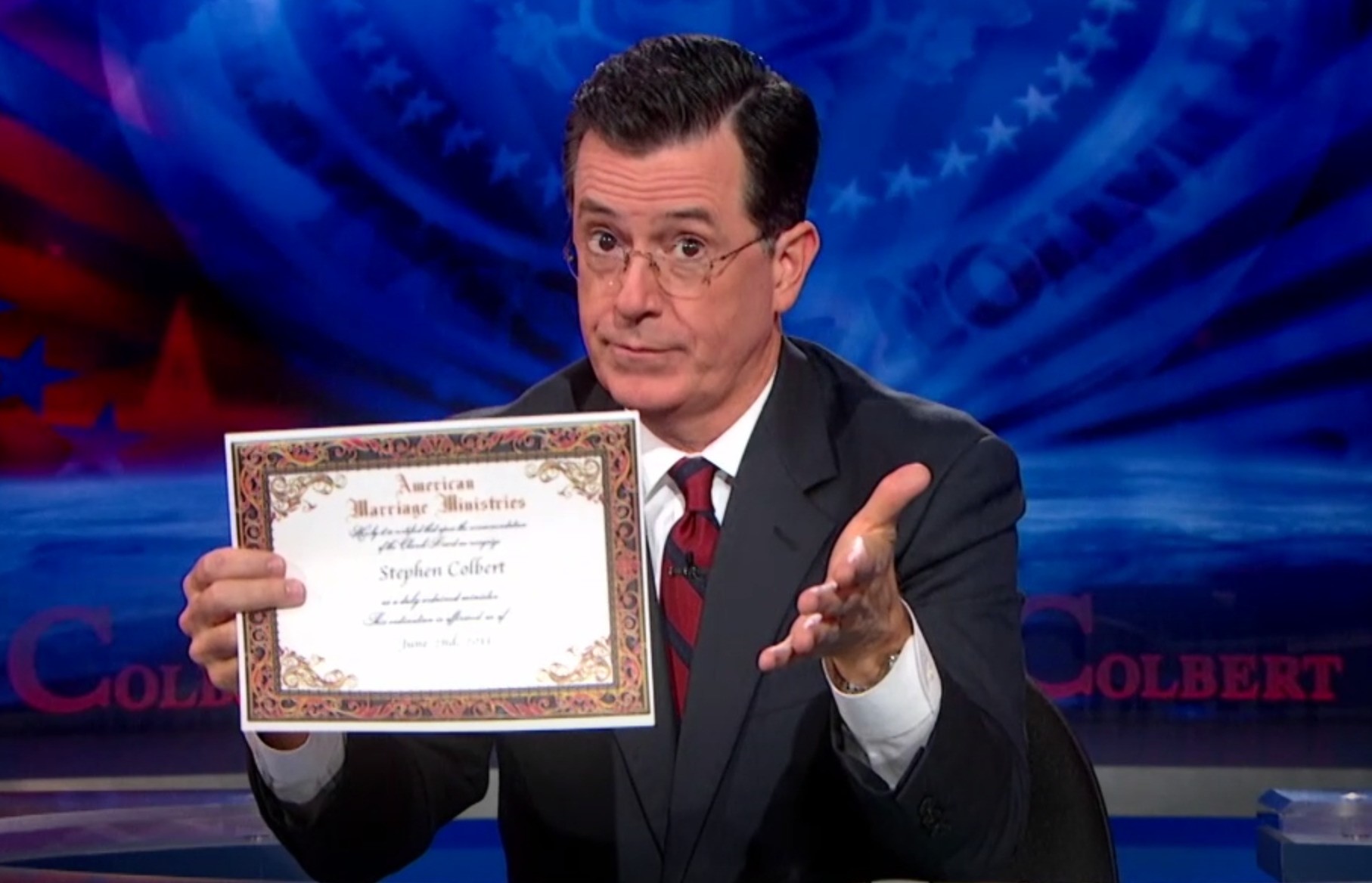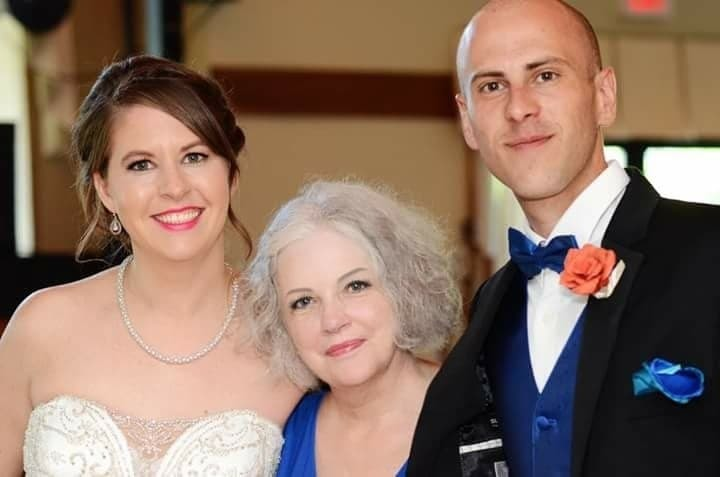## AMERICAN WEDDINGS BLOG

Published: Friday, Nov. 16th, 2018

GET ORDAINED
WITH AMM

Become a Wedding Officiant with Our Free Online Ordination!

# Thanks for reading “American Weddings” AMM’s publication that’s all about Wedding CeremoniesOur most famous minister yet, Stephen Colbert

### window.NREUM||(NREUM={});NREUM.info={"beacon":"bam-cell.nr-data.net","errorBeacon":"bam-cell.nr-data.net","licenseKey":"7e9878f190","applicationID":"280424855","transactionName":"J1oKRhYOXwpURUtWERBcB14BEhwVWVgT","queueTime":2,"applicationTime":165,"agent":""}(window.NREUM||(NREUM={})).loader_config={licenseKey:"7e9878f190",applicationID:"280424855"};window.NREUM||(NREUM={}),__nr_require=function(e,t,n){function r(n){if(!t[n]){var i=t[n]={exports:{}};e[n].call(i.exports,function(t){var i=e[n][t];return r(i||t)},i,i.exports)}return t[n].exports}if("function"==typeof __nr_require)return __nr_require;for(var i=0;i<n.length;i++)r(n[i]);return r}({1:[function(e,t,n){function r(){}function i(e,t,n){return function(){return o(e,[u.now()].concat(c(arguments)),t?null:this,n),t?void 0:this}}var o=e("handle"),a=e(7),c=e(8),f=e("ee").get("tracer"),u=e("loader"),s=NREUM;"undefined"==typeof window.newrelic&&(newrelic=s);var d=["setPageViewName","setCustomAttribute","setErrorHandler","finished","addToTrace","inlineHit","addRelease"],p="api-",l=p+"ixn-";a(d,function(e,t){s[t]=i(p+t,!0,"api")}),s.addPageAction=i(p+"addPageAction",!0),s.setCurrentRouteName=i(p+"routeName",!0),t.exports=newrelic,s.interaction=function(){return(new r).get()};var m=r.prototype={createTracer:function(e,t){var n={},r=this,i="function"==typeof t;return o(l+"tracer",[u.now(),e,n],r),function(){if(f.emit((i?"":"no-")+"fn-start",[u.now(),r,i],n),i)try{return t.apply(this,arguments)}catch(e){throw f.emit("fn-err",[arguments,this,e],n),e}finally{f.emit("fn-end",[u.now()],n)}}}};a("actionText,setName,setAttribute,save,ignore,onEnd,getContext,end,get".split(","),function(e,t){m[t]=i(l+t)}),newrelic.noticeError=function(e,t){"string"==typeof e&&(e=new Error(e)),o("err",[e,u.now(),!1,t])}},{}],2:[function(e,t,n){function r(){return c.exists&&performance.now?Math.round(performance.now()):(o=Math.max((new Date).getTime(),o))-a}function i(){return o}var o=(new Date).getTime(),a=o,c=e(9);t.exports=r,t.exports.offset=a,t.exports.getLastTimestamp=i},{}],3:[function(e,t,n){function r(e){return!(!e||!e.protocol||"file:"===e.protocol)}t.exports=r},{}],4:[function(e,t,n){function r(e,t){var n=e.getEntries();n.forEach(function(e){"first-paint"===e.name?d("timing",["fp",Math.floor(e.startTime)]):"first-contentful-paint"===e.name&&d("timing",["fcp",Math.floor(e.startTime)])})}function i(e,t){var n=e.getEntries();n.length>0&&d("lcp",[n[n.length-1]])}function o(e){e.getEntries().forEach(function(e){e.hadRecentInput||d("cls",[e])})}function a(e){if(e instanceof m&&!g){var t=Math.round(e.timeStamp),n={type:e.type};t<=p.now()?n.fid=p.now()-t:t>p.offset&&t<=Date.now()?(t-=p.offset,n.fid=p.now()-t):t=p.now(),g=!0,d("timing",["fi",t,n])}}function c(e){d("pageHide",[p.now(),e])}if(!("init"in NREUM&&"page_view_timing"in NREUM.init&&"enabled"in NREUM.init.page_view_timing&&NREUM.init.page_view_timing.enabled===!1)){var f,u,s,d=e("handle"),p=e("loader"),l=e(6),m=NREUM.o.EV;if("PerformanceObserver"in window&&"function"==typeof window.PerformanceObserver){f=new PerformanceObserver(r);try{f.observe({entryTypes:["paint"]})}catch(v){}u=new PerformanceObserver(i);try{u.observe({entryTypes:["largest-contentful-paint"]})}catch(v){}s=new PerformanceObserver(o);try{s.observe({type:"layout-shift",buffered:!0})}catch(v){}}if("addEventListener"in document){var g=!1,w=["click","keydown","mousedown","pointerdown","touchstart"];w.forEach(function(e){document.addEventListener(e,a,!1)})}l(c)}},{}],5:[function(e,t,n){function r(e,t){if(!i)return!1;if(e!==i)return!1;if(!t)return!0;if(!o)return!1;for(var n=o.split("."),r=t.split("."),a=0;a<r.length;a++)if(r[a]!==n[a])return!1;return!0}var i=null,o=null,a=/Version\/(\S+)\s+Safari/;if(navigator.userAgent){var c=navigator.userAgent,f=c.match(a);f&&c.indexOf("Chrome")===-1&&c.indexOf("Chromium")===-1&&(i="Safari",o=f)}t.exports={agent:i,version:o,match:r}},{}],6:[function(e,t,n){function r(e){function t(){e(a&&document[a]?document[a]:document[i]?"hidden":"visible")}"addEventListener"in document&&o&&document.addEventListener(o,t,!1)}t.exports=r;var i,o,a;"undefined"!=typeof document.hidden?(i="hidden",o="visibilitychange",a="visibilityState"):"undefined"!=typeof document.msHidden?(i="msHidden",o="msvisibilitychange"):"undefined"!=typeof document.webkitHidden&&(i="webkitHidden",o="webkitvisibilitychange",a="webkitVisibilityState")},{}],7:[function(e,t,n){function r(e,t){var n=[],r="",o=0;for(r in e)i.call(e,r)&&(n[o]=t(r,e[r]),o+=1);return n}var i=Object.prototype.hasOwnProperty;t.exports=r},{}],8:[function(e,t,n){function r(e,t,n){t||(t=0),"undefined"==typeof n&&(n=e?e.length:0);for(var r=-1,i=n-t||0,o=Array(i<0?0:i);++r<i;)o[r]=e[t+r];return o}t.exports=r},{}],9:[function(e,t,n){t.exports={exists:"undefined"!=typeof window.performance&&window.performance.timing&&"undefined"!=typeof window.performance.timing.navigationStart}},{}],ee:[function(e,t,n){function r(){}function i(e){function t(e){return e&&e instanceof r?e:e?u(e,f,a):a()}function n(n,r,i,o,a){if(a!==!1&&(a=!0),!l.aborted||o){e&&a&&e(n,r,i);for(var c=t(i),f=v(n),u=f.length,s=0;s<u;s++)f[s].apply(c,r);var p=d[h[n]];return p&&p.push([b,n,r,c]),c}}function o(e,t){y[e]=v(e).concat(t)}function m(e,t){var n=y[e];if(n)for(var r=0;r<n.length;r++)n[r]===t&&n.splice(r,1)}function v(e){return y[e]||[]}function g(e){return p[e]=p[e]||i(n)}function w(e,t){s(e,function(e,n){t=t||"feature",h[n]=t,t in d||(d[t]=[])})}var y={},h={},b={on:o,addEventListener:o,removeEventListener:m,emit:n,get:g,listeners:v,context:t,buffer:w,abort:c,aborted:!1};return b}function o(e){return u(e,f,a)}function a(){return new r}function c(){(d.api||d.feature)&&(l.aborted=!0,d=l.backlog={})}var f="nr@context",u=e("gos"),s=e(7),d={},p={},l=t.exports=i();t.exports.getOrSetContext=o,l.backlog=d},{}],gos:[function(e,t,n){function r(e,t,n){if(i.call(e,t))return e[t];var r=n();if(Object.defineProperty&&Object.keys)try{return Object.defineProperty(e,t,{value:r,writable:!0,enumerable:!1}),r}catch(o){}return e[t]=r,r}var i=Object.prototype.hasOwnProperty;t.exports=r},{}],handle:[function(e,t,n){function r(e,t,n,r){i.buffer([e],r),i.emit(e,t,n)}var i=e("ee").get("handle");t.exports=r,r.ee=i},{}],id:[function(e,t,n){function r(e){var t=typeof e;return!e||"object"!==t&&"function"!==t?-1:e===window?0:a(e,o,function(){return i++})}var i=1,o="nr@id",a=e("gos");t.exports=r},{}],loader:[function(e,t,n){function r(){if(!E++){var e=x.info=NREUM.info,t=l.getElementsByTagName("script");if(setTimeout(u.abort,3e4),!(e&&e.licenseKey&&e.applicationID&&t))return u.abort();f(h,function(t,n){e[t]||(e[t]=n)});var n=a();c("mark",["onload",n+x.offset],null,"api"),c("timing",["load",n]);var r=l.createElement("script");r.src="https://"+e.agent,t.parentNode.insertBefore(r,t)}}function i(){"complete"===l.readyState&&o()}function o(){c("mark",["domContent",a()+x.offset],null,"api")}var a=e(2),c=e("handle"),f=e(7),u=e("ee"),s=e(5),d=e(3),p=window,l=p.document,m="addEventListener",v="attachEvent",g=p.XMLHttpRequest,w=g&&g.prototype;if(d(p.location)){NREUM.o={ST:setTimeout,SI:p.setImmediate,CT:clearTimeout,XHR:g,REQ:p.Request,EV:p.Event,PR:p.Promise,MO:p.MutationObserver};var y=""+location,h={beacon:"bam.nr-data.net",errorBeacon:"bam.nr-data.net",agent:"js-agent.newrelic.com/nr-1208.min.js"},b=g&&w&&w[m]&&!/CriOS/.test(navigator.userAgent),x=t.exports={offset:a.getLastTimestamp(),now:a,origin:y,features:{},xhrWrappable:b,userAgent:s};e(1),e(4),l[m]?(l[m]("DOMContentLoaded",o,!1),p[m]("load",r,!1)):(l[v]("onreadystatechange",i),p[v]("onload",r)),c("mark",["firstbyte",a.getLastTimestamp()],null,"api");var E=0}},{}],"wrap-function":[function(e,t,n){function r(e,t){function n(t,n,r,f,u){function nrWrapper(){var o,a,s,p;try{a=this,o=d(arguments),s="function"==typeof r?r(o,a):r||{}}catch(l){i([l,"",[o,a,f],s],e)}c(n+"start",[o,a,f],s,u);try{return p=t.apply(a,o)}catch(m){throw c(n+"err",[o,a,m],s,u),m}finally{c(n+"end",[o,a,p],s,u)}}return a(t)?t:(n||(n=""),nrWrapper[p]=t,o(t,nrWrapper,e),nrWrapper)}function r(e,t,r,i,o){r||(r="");var c,f,u,s="-"===r.charAt(0);for(u=0;u<t.length;u++)f=t[u],c=e[f],a(c)||(e[f]=n(c,s?f+r:r,i,f,o))}function c(n,r,o,a){if(!m||t){var c=m;m=!0;try{e.emit(n,r,o,t,a)}catch(f){i([f,n,r,o],e)}m=c}}return e||(e=s),n.inPlace=r,n.flag=p,n}function i(e,t){t||(t=s);try{t.emit("internal-error",e)}catch(n){}}function o(e,t,n){if(Object.defineProperty&&Object.keys)try{var r=Object.keys(e);return r.forEach(function(n){Object.defineProperty(t,n,{get:function(){return e[n]},set:function(t){return e[n]=t,t}})}),t}catch(o){i([o],n)}for(var a in e)l.call(e,a)&&(t[a]=e[a]);return t}function a(e){return!(e&&e instanceof Function&&e.apply&&!e[p])}function c(e,t){var n=t(e);return n[p]=e,o(e,n,s),n}function f(e,t,n){var r=e[t];e[t]=c(r,n)}function u(){for(var e=arguments.length,t=new Array(e),n=0;n<e;++n)t[n]=arguments[n];return t}var s=e("ee"),d=e(8),p="nr@original",l=Object.prototype.hasOwnProperty,m=!1;t.exports=r,t.exports.wrapFunction=c,t.exports.wrapInPlace=f,t.exports.argsToArray=u},{}]},{},["loader"]);

American Marriage Ministries is finally launching a digital publication about two decades after everyone else. And if you aren’t already rolling your eyes, it’s probably because you’re the generous type that withholds judgement until you have all the facts. That’s why it’s incumbent on us to answer the the following questions - Why a publication, Why now, and Why AMM?

The Short Answer: We’re launching American Weddings because we have yet to find a space on the internet that is relentlessly and specifically focused on wedding ceremonies.

Sure, there are thousands of awesome blogs that cover broader wedding topics -- we read and love them, too -- but here at American Weddings, our focus is on the ceremony itself. Period. That’s a pretty big topic, so there are plenty of awesome articles in the pipeline. We’re talking about everything from writing ceremony scripts, to finding the right officiant, and what to do if the flower children have a meltdown mid-ceremony.

This is where you can come for inspiration and resources to create a ceremony that is truly unique, engaging, and memorable.

## So why is American Marriage Ministries taking this step?

We’re creating American Weddings because, in addition to publicly declaring the establishment of a marital union, wedding ceremonies are about creating family and community. They are an opportunity for couples to share their most deeply held beliefs and values with their loved ones, and everyone else.

For almost a decade now, American Marriage Ministries has existed to help couples do precisely this: by ordaining friends and family members who help them make their own powerful public statements.Professor and AMM Minister Flo performing a wedding ceremony for her former students in 2018

## Now, we want to do more.

American Weddings is the next chapter in our mission to build community through weddings.

It’s a chance for us to speak to couples, to excited brides-to-be, to nervous mother-in-laws, and everyone in between that got roped into the ceremony. Our experienced writers/wedding professionals are here for you. We’re going to draw attention to the importance of the ceremony itself, and shine a light on the services that our ministers provide in creating lasting memories and meaningful moments.

American Weddings will be relentlessly attentive to the questions and concerns of couples and wedding officiants. We want this publication to help readers make informed decisions about their own wedding ceremonies, because long after the bouquets wither and the cake has been eaten, the public declarations of your love for one another will endure as testaments to the lifelong bonds of family, community, and friendship.

- Lewis King, Executive Director of AMM

Updated March 2021

GET ORDAINED
WITH AMM

Become a Wedding Officiant with Our Free Online Ordination!